## Python Tutorial

Python HOME Python Intro Python Get Started Python Syntax Python Comments Python Variables Python Data Types Python Numbers Python Casting Python Strings Python Booleans Python Operators Python Lists Python Tuples Python Sets Python Dictionaries Python If...Else Python While Loops Python For Loops Python Functions Python Lambda Python Arrays Python Classes/Objects Python Inheritance Python Iterators Python Scope Python Modules Python Dates Python Math Python JSON Python RegEx Python PIP Python Try...Except Python User Input Python String Formatting

## File Handling

Python File Handling Python Read Files Python Write/Create Files Python Delete Files

## Python Modules

NumPy Tutorial Pandas Tutorial SciPy Tutorial Django Tutorial

## Python Matplotlib

Matplotlib Intro Matplotlib Get Started Matplotlib Pyplot Matplotlib Plotting Matplotlib Markers Matplotlib Line Matplotlib Labels Matplotlib Grid Matplotlib Subplot Matplotlib Scatter Matplotlib Bars Matplotlib Histograms Matplotlib Pie Charts

## Machine Learning

Getting Started Mean Median Mode Standard Deviation Percentile Data Distribution Normal Data Distribution Scatter Plot Linear Regression Polynomial Regression Multiple Regression Scale Train/Test Decision Tree Confusion Matrix Hierarchical Clustering Logistic Regression Grid Search Categorical Data K-means Bootstrap Aggregation Cross Validation AUC - ROC Curve K-nearest neighbors

## Python MySQL

MySQL Get Started MySQL Create Database MySQL Create Table MySQL Insert MySQL Select MySQL Where MySQL Order By MySQL Delete MySQL Drop Table MySQL Update MySQL Limit MySQL Join

## Python MongoDB

MongoDB Get Started MongoDB Create Database MongoDB Create Collection MongoDB Insert MongoDB Find MongoDB Query MongoDB Sort MongoDB Delete MongoDB Drop Collection MongoDB Update MongoDB Limit

## Python Reference

Python Overview Python Built-in Functions Python String Methods Python List Methods Python Dictionary Methods Python Tuple Methods Python Set Methods Python File Methods Python Keywords Python Exceptions Python Glossary

## Module Reference

Random Module Requests Module Statistics Module Math Module cMath Module

## Python How To

Remove List Duplicates Reverse a String Add Two Numbers

## Python Examples

Python Examples Python Compiler Python Exercises Python Quiz Python Certificate

# Machine Learning - Decision Tree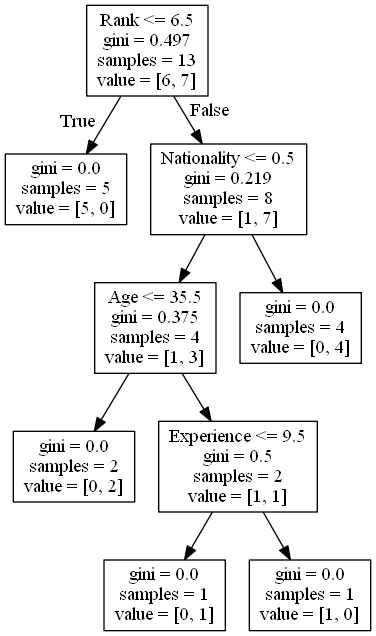## Decision Tree

In this chapter we will show you how to make a "Decision Tree". A Decision Tree is a Flow Chart, and can help you make decisions based on previous experience.

In the example, a person will try to decide if he/she should go to a comedy show or not.

Luckily our example person has registered every time there was a comedy show in town, and registered some information about the comedian, and also registered if he/she went or not.

 Age Experience Rank Nationality Go 36 10 9 UK NO 42 12 4 USA NO 23 4 6 N NO 52 4 4 USA NO 43 21 8 USA YES 44 14 5 UK NO 66 3 7 N YES 35 14 9 UK YES 52 13 7 N YES 35 5 9 N YES 24 3 5 USA NO 18 3 7 UK YES 45 9 9 UK YES

Now, based on this data set, Python can create a decision tree that can be used to decide if any new shows are worth attending to.

## Learn to Filter Data in Python Like a Data AnalystTry a hands-on training sessions with step-by-step guidance from an expert. Try the guided project made in collaboration with Coursera now!

Get started

## How Does it Work?

First, read the dataset with pandas:

### Example

Read and print the data set:

import pandas

print(df)

To make a decision tree, all data has to be numerical.

We have to convert the non numerical columns 'Nationality' and 'Go' into numerical values.

Pandas has a `map()` method that takes a dictionary with information on how to convert the values.

`{'UK': 0, 'USA': 1, 'N': 2}`

Means convert the values 'UK' to 0, 'USA' to 1, and 'N' to 2.

### Example

Change string values into numerical values:

d = {'UK': 0, 'USA': 1, 'N': 2}
df['Nationality'] = df['Nationality'].map(d)
d = {'YES': 1, 'NO': 0}
df['Go'] = df['Go'].map(d)

print(df)

Then we have to separate the feature columns from the target column.

The feature columns are the columns that we try to predict from, and the target column is the column with the values we try to predict.

### Example

`X` is the feature columns, `y` is the target column:

features = ['Age', 'Experience', 'Rank', 'Nationality']

X = df[features]
y = df['Go']

print(X)
print(y)

Now we can create the actual decision tree, fit it with our details. Start by importing the modules we need:

### Example

Create and display a Decision Tree:

import pandas
from sklearn import tree
from sklearn.tree import DecisionTreeClassifier
import matplotlib.pyplot as plt

d = {'UK': 0, 'USA': 1, 'N': 2}
df['Nationality'] = df['Nationality'].map(d)
d = {'YES': 1, 'NO': 0}
df['Go'] = df['Go'].map(d)

features = ['Age', 'Experience', 'Rank', 'Nationality']

X = df[features]
y = df['Go']

dtree = DecisionTreeClassifier()
dtree = dtree.fit(X, y)

tree.plot_tree(dtree, feature_names=features)

## Result Explained

The decision tree uses your earlier decisions to calculate the odds for you to wanting to go see a comedian or not.

Let us read the different aspects of the decision tree: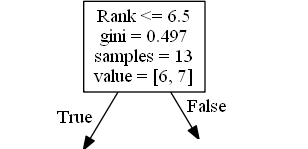### Rank

`Rank <= 6.5` means that every comedian with a rank of 6.5 or lower will follow the `True` arrow (to the left), and the rest will follow the `False` arrow (to the right).

`gini = 0.497` refers to the quality of the split, and is always a number between 0.0 and 0.5, where 0.0 would mean all of the samples got the same result, and 0.5 would mean that the split is done exactly in the middle.

`samples = 13` means that there are 13 comedians left at this point in the decision, which is all of them since this is the first step.

`value = [6, 7]` means that of these 13 comedians, 6 will get a "NO", and 7 will get a "GO".

### Gini

There are many ways to split the samples, we use the GINI method in this tutorial.

The Gini method uses this formula:

`Gini = 1 - (x/n)2 + (y/n)2`

Where `x` is the number of positive answers("GO"), `n` is the number of samples, and `y` is the number of negative answers ("NO"), which gives us this calculation:

`1 - (7 / 13)2 + (6 / 13)2 = 0.497`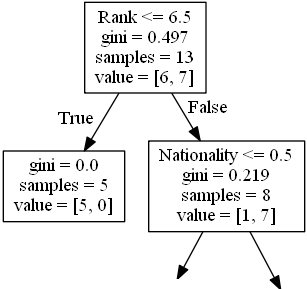The next step contains two boxes, one box for the comedians with a 'Rank' of 6.5 or lower, and one box with the rest.

### True - 5 Comedians End Here:

`gini = 0.0` means all of the samples got the same result.

`samples = 5` means that there are 5 comedians left in this branch (5 comedian with a Rank of 6.5 or lower).

`value = [5, 0]` means that 5 will get a "NO" and 0 will get a "GO".

### Nationality

`Nationality <= 0.5` means that the comedians with a nationality value of less than 0.5 will follow the arrow to the left (which means everyone from the UK, ), and the rest will follow the arrow to the right.

`gini = 0.219` means that about 22% of the samples would go in one direction.

`samples = 8` means that there are 8 comedians left in this branch (8 comedian with a Rank higher than 6.5).

`value = [1, 7]` means that of these 8 comedians, 1 will get a "NO" and 7 will get a "GO".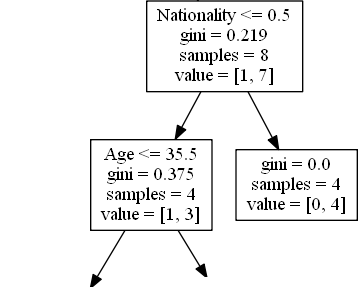### Age

`Age <= 35.5` means that comedians at the age of 35.5 or younger will follow the arrow to the left, and the rest will follow the arrow to the right.

`gini = 0.375` means that about 37,5% of the samples would go in one direction.

`samples = 4` means that there are 4 comedians left in this branch (4 comedians from the UK).

`value = [1, 3]` means that of these 4 comedians, 1 will get a "NO" and 3 will get a "GO".

### False - 4 Comedians End Here:

`gini = 0.0` means all of the samples got the same result.

`samples = 4` means that there are 4 comedians left in this branch (4 comedians not from the UK).

`value = [0, 4]` means that of these 4 comedians, 0 will get a "NO" and 4 will get a "GO".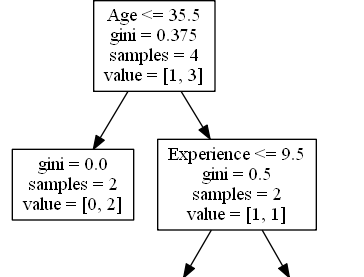### True - 2 Comedians End Here:

`gini = 0.0` means all of the samples got the same result.

`samples = 2` means that there are 2 comedians left in this branch (2 comedians at the age 35.5 or younger).

`value = [0, 2]` means that of these 2 comedians, 0 will get a "NO" and 2 will get a "GO".

### Experience

`Experience <= 9.5` means that comedians with 9.5 years of experience, or less, will follow the arrow to the left, and the rest will follow the arrow to the right.

`gini = 0.5` means that 50% of the samples would go in one direction.

`samples = 2` means that there are 2 comedians left in this branch (2 comedians older than 35.5).

`value = [1, 1]` means that of these 2 comedians, 1 will get a "NO" and 1 will get a "GO".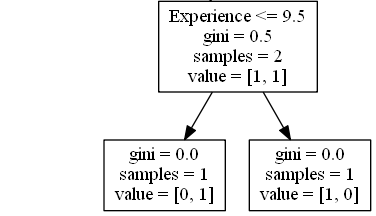### True - 1 Comedian Ends Here:

`gini = 0.0` means all of the samples got the same result.

`samples = 1` means that there is 1 comedian left in this branch (1 comedian with 9.5 years of experience or less).

`value = [0, 1]` means that 0 will get a "NO" and 1 will get a "GO".

### False - 1 Comedian Ends Here:

`gini = 0.0` means all of the samples got the same result.

`samples = 1` means that there is 1 comedians left in this branch (1 comedian with more than 9.5 years of experience).

`value = [1, 0]` means that 1 will get a "NO" and 0 will get a "GO".

## Predict Values

We can use the Decision Tree to predict new values.

Example: Should I go see a show starring a 40 years old American comedian, with 10 years of experience, and a comedy ranking of 7?

### Example

Use predict() method to predict new values:

print(dtree.predict([[40, 10, 7, 1]]))

### Example

What would the answer be if the comedy rank was 6?

print(dtree.predict([[40, 10, 6, 1]]))

### Different Results

You will see that the Decision Tree gives you different results if you run it enough times, even if you feed it with the same data.

That is because the Decision Tree does not give us a 100% certain answer. It is based on the probability of an outcome, and the answer will vary.